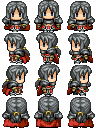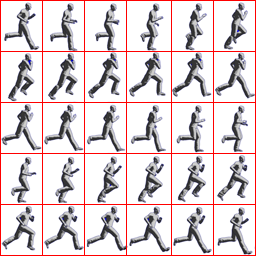## Sprite

##### A very lightweight library to handle sprites on HTML Canvas#### Installation

You can easily get it from bower

``\$ bower install sprite``

Or get it from cdnjs

``<script src="//cdnjs.cloudflare.com/ajax/libs/sprite-js/0.1.0/sprite.min.js"></script>``

#### Usage

Basically you can build a sprite by instantiating the Sprite class

``var sprite = new Sprite(options)``

You should pass some arguments to Sprite, so that it can know:

``````
// (r) = required
// (d N) = defaults to N
var sprite = new Sprite({
canvas: document.getElementById('canvas'), // Where to draw? (r)
image: document.getElementById('image'),   // What image to use? (r)
rows: 4,           // How many rows are there in the image?
columns: 3,        // How many columns are there in the image?
rowIndex: 0,       // Where to start drawing the row? (d 0)
columnIndex: 0,    // Where to start drawing the column? (d 0)
rowFrequency: 1,   // After how many draw cycles row is updated? (d 0)
columnFrequency: 1,// After how many draw cycles column is updated? (d 0)
rowCircular: true,    // true  -> 0, 1, 2, 0, 1, 2, 0, 1, 2... (d fls)
columnCircular: true, // false -> 0, 1, 2, 1, 0, 1, 2, 1, 0... (d fls)
rowDirection: 4,   // row increment (d 1)
columnDirection: 4,// column increment (d 1)
width: 100,        // Drawing width (d (image.width / columns))
height: 100,       // Drawing height (d (image.height / rows))
})
``````

After that, you can draw your sprite

``````
// x, y are the coordinates where the sprite should be drawed on the canvas
sprite.draw(x, y)
``````

And you can also change the sprite properties dynamically

``````
sprite.rowFrequency = 20;
sprite.rowIndex = 1;
(...)
``````

#### Examples

All of the codes above use a chunk of code like this to render the code on loop:

``````
var canvas = sprite.canvas;
function loop() {
canvas.getContext('2d').clearRect(0, 0, canvas.width, canvas.height);
sprite.draw(0, 0);
}
setInterval(loop, 200);
``````Source: ちびキャラツクール / ファミ通
``````
var sprite = new Sprite({
canvas: document.getElementById('canvas'),
image: document.getElementById('image'),

});
````````````
var sprite = new Sprite({
canvas: document.getElementById('canvas'),
image: document.getElementById('image'),

});
``````